T-LAB PLUS 2022 - ON-LINE HELP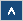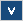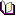T-LABIntroduction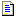What T-LAB does and what it enables us to doRequirements and PerformancesCorpus PreparationCorpus PreparationStructural CriteriaFormal CriteriaFileImport a single file...Prepare a Corpus (Corpus Builder)Open an existing projectSettingsAutomatic and Customized SettingsDictionary BuildingCo-occurrence AnalysisWord AssociationsCo-Word Analysis and Concept MappingComparison between Word pairsSequence and Network AnalysisConcordancesThematic AnalysisThematic Analysis of Elementary ContextsModeling of Emerging ThemesThematic Document ClassificationDictionary-Based ClassificationTexts and Discourses as Dynamic SystemsComparative AnalysisSpecificity AnalysisCorrespondence AnalysisMultiple Correspondence AnalysisCluster AnalysisSingular Value DecompositionLexical ToolsText Screening / DisambiguationsCorpus VocabularyStop-Word ListMulti-Word ListWord SegmentationOther ToolsVariable ManagerAdvanced Corpus SearchClassification of New DocumentsKey Contexts of Thematic WordsExport Custom TablesEditorImport-Export Identifiers listGlossaryAnalysis UnitAssociation IndexesChi-SquareCluster AnalysisCodingContext UnitCorpus and SubsetsCorrespondence AnalysisData TableDisambiguationDictionaryElementary ContextFrequency ThresholdGraph MakerHomographIDnumberIsotopyKey-Word (Key-Term)LemmatizationLexical UnitLexie and LexicalizationMarkov ChainMDSMultiwordsN-gramsNaïve BayesNormalizationOccurrences and Co-occurrencesPoles of FactorsPrimary DocumentProfileSpecificityStop Word ListTest ValueThematic NucleusTF-IDFVariables and CategoriesWords and LemmasBibliography
www.tlab.it

Singular Value Decomposition

The Singular Value Decomposition (SVD - see Wikipedia https://en.wikipedia.org/wiki/Singular-value_decomposition) is a technique for dimensionality reduction, which - in Text Mining - can be used for discovering the latent dimensions (or components) which determine semantic similarities between words (i.e. lexical units) or between documents (i.e. context units).

T-LAB allows us to perform a Singular Value Decomposition of three types of data tables. In the first case (see 'A' below), the data table is a co-occurrence matrix whose rows and columns are key-terms. In the second case (see 'B' below), a data table elementary contexts x key-terms will be filled with presence/absence values (i.e. '1' and '0'). In the third case (see 'C' below), a data table documents x key-terms will be filled with occurrence values.

N.B.: Please note that, when analysing co-occurrence matrix whose rows and columns are key-terms (see 'A' below), T-LAB provides high-quality dense vectors (i.e. word embeddings).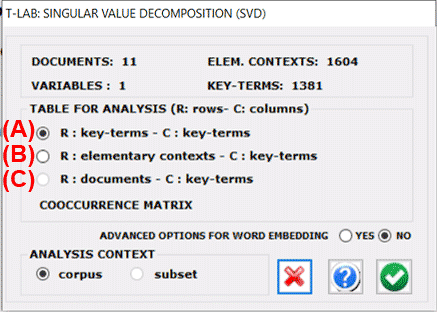The analysis procedure consists of the following steps:
1 - construction of the data table to be analysed (up to 300,000 rows x 5,000 columns);
2 - TF-IDF normalization and scaling of row vectors to unit length (Euclidean norm);
3 - extraction of first 20 'latent dimensions' through the Lanczos algorithm.
N.B.:
-
In the case of co-occurrence matrix (see 'A' above), data normalization is performed through the cosine measure.
- When the advanced options for word embedding are selected, T-LAB computes PPMI values (Positive Pointwise Mutual Information) and makes it possible to use the first 50 dimensions of the SVD.

The analysis results are displayed in tables and charts.

In detail:

Two tables - the rows of which can be either lexical units or context units - have as many columns as the extracted dimensions.

In the case of the LEMMAS (i.e. lexical units) table, a further column is displayed, in which the importance scores are reported (see below).N.B.: The importance score of each lemma is computed by summing the absolute values of its first 20 coordinates (i.e. the eigenvectors), each one multiplied by its corresponding eigenvalue.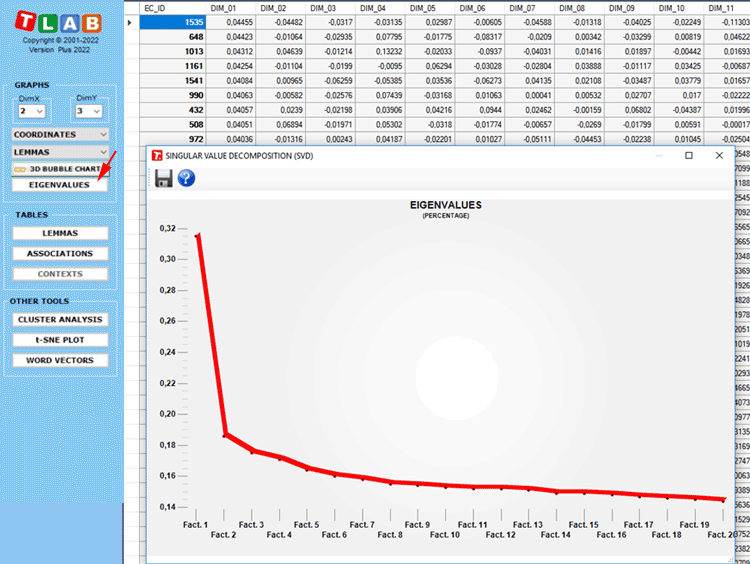Any table can be sorted in ascending or descending order by clicking on any column header.
In order to export any table, just use the right click of the mouse when data are displayed.
Please note that, the first time such a table is exported, the Eigenvalues are also exported. This way the user is allowed to evaluate the relative weight of each dimension, that is the percentage of variance explained by each one of them.

By clicking the Associations button (see below), a further table is displayed with the similarity measures (i.e. cosine coefficients) of each word. Moreover, when any row of such a table is clicked, a graph is displayed with the corresponding data.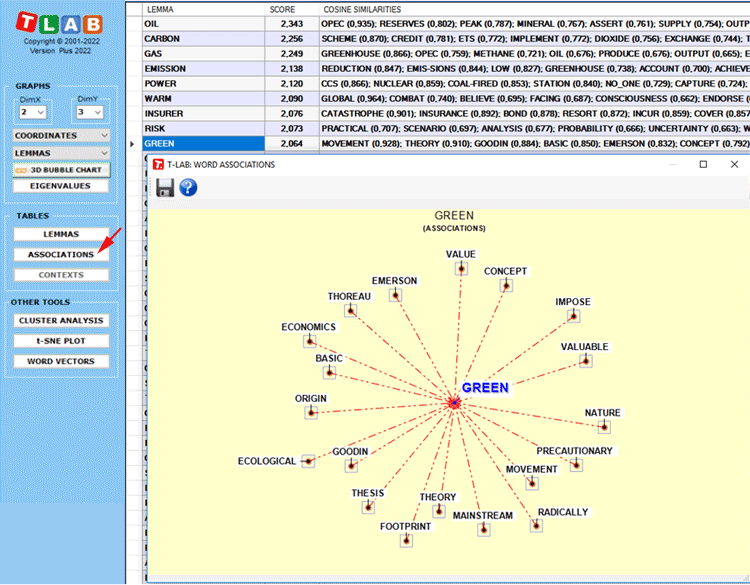The main charts shows the relationships between the key-terms (i.e. lemmas) on the selected dimensions (see below).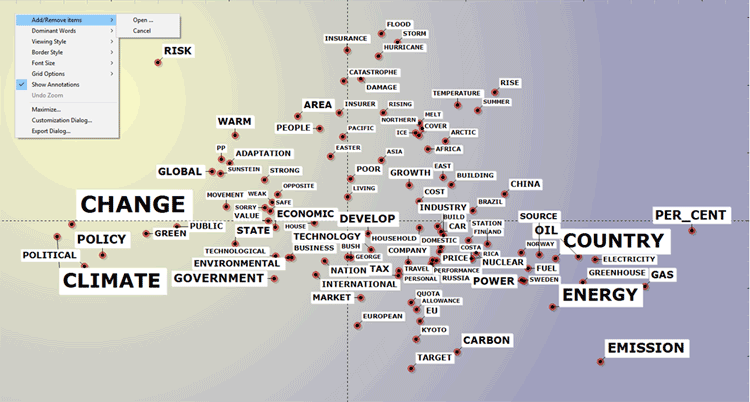By default, the above chart includes the 100 most important lemmas. However the user is allowed to customize both the number of lemmas and the chart characteristics.